History of "0" From Zero to Hero. Watch the storyYodi Supriyadi
Saturday, February 19, 2022

# History of "0" From Zero to Hero. Watch the story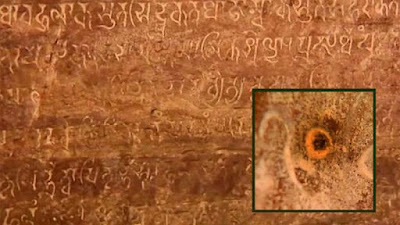Ancient Artifact number 0

Purwakarta - The number 0 (zero) is a number to represent a non-existence. Almost every day, the number zero is always present and inherent in human life. For example, when you want to write down a cellphone number, calculate buying and selling transactions, to pay electricity bills, this number cannot be separated from the role of this number.

But do you know, apparently hundreds of years ago humans only knew 9 numbers, namely 1, 2, 3, 4, 5, 6, 7, 8, and 9? Zero is the most recently discovered number.

The concept of the number zero was used at least in the 400th century BC by Babylonian mathematicians. At that time, zero was not written with the number '0', but with the symbol "only.".

How is the journey of the number 0 from the past until now?. This is a brief history of the journey of the number 0.

1. Used as a barrier by the Babylonians

The number zero was already known and used by the Babylonians who lived in the Mezopotamia Valley around 300 BC ago, guys. Initially they used the symbol of two slashes to represent an empty column of numbers,
Boundary Image used by the Babylonians

From research on Babylonian stone tablets, it seems that the symbol for the number zero "was originally used in Babylon to distinguish the number in question. For example, such as distinguishing the numbers 216 and 21"6 (2106), as quoted from Marina Toma's research, "A History of Zero" in the Journal of Science and Arts.

The concept of the number zero was also found on tablets at Kish, an ancient Mesopotamian city in eastern Babylon that is now part of Iraq. This tablet apparently comes from an earlier period, which is around 700 BC (BC). Slightly different from the Babylonian tablets, the zero symbol in Kish uses triple quotes.

However, both artifacts show that zero is never used at the end of a number, so it is always in the middle of a number. For example, there is the number 21"6, but no number 216".

Even though they have known and used the number zero, unfortunately they still haven't studied its properties. So the number zero still does not have a stand-alone numeric value.

2. Used for calendar calculations by the Maya in America

600 years later, the number zero was discovered in the Mayan civilization which was 12 miles away from the Babylonians. The people who live in the Americas developed the number zero for their calendar calculation system. Zero is represented by the eye symbol.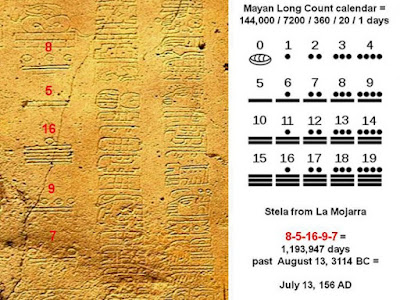Mayan Calendar System

Interestingly, although they are also known to be very good at math, they don't use these zeros to calculate equations. Maybe this zero has not been considered something important this time, huh.

3. Just becoming a number that has its own value in India

The concept of zero only emerged and developed in India around the year 458 AD. Uniquely, the concept of zero appeared for the first time in the form of pronunciation in mathematical equations, poetry, or singing. Zero in Sanskrit is called "sunya", symbolized by several different words, such as "space", "void", or "space".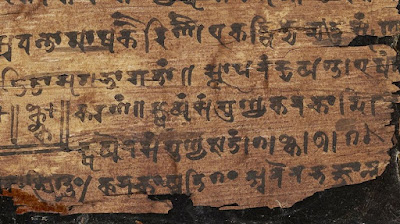Ancient Relic

Furthermore, it was not until AD 628 that an astronomer and mathematician named Brahmagupta developed its own properties for zero. This Indian mathematician showed that the number zero could serve to separate positive and negative numbers. In addition, he also developed mathematical operations such as addition and subtraction, as well as multiplication and division using the number zero. So now it can be seen that 2 + (-2) = 0.

The Indians write the number zero with the symbol of a dot. The full stop is also used as an unknown symbol, which is now symbolized as 'x'. The number zero in India at that time was called kha, but it did not have the '0' symbol as it does today. At this time, for the first time in world history, the number zero has a value that stands alone.

The Indians write the number zero with the symbol of a dot. The full stop is also used as an unknown symbol, which is now symbolized as 'x'. The number zero in India at that time was called kha, but it did not have the '0' symbol as it does today. At this time, for the first time in world history, the number zero has a value that stands alone.

The use of the number 0 in numbers was initiated by an Indian mathematician named Brahmagupta (598-670 BC). He stated that:
• 0 + negative number = negative number; and negative numbers - 0 = negative numbers
• 0 + positive number = positive number; and positive number - 0 = positive number
• 0 + 0 = 0 and 0 - 0 = 0
• 0 - negative number = positive number
• 0 - positive number = negative number
Number divided by 0 is undefined and has no arithmetic meaning.

4. From Arabic widely introduced by a Muslim intellectual named Al-Khwarizmi

One of the mathematicians from Persia, named Muhammad Ibn Musa Al-Khwarizm, who is known today as Al-Khwarizmi.

Al-Khwarizmi is the inventor of the number zero and the father of world algebra. Al-Khwarizmi suggested that a small circle should be used in calculations if there is no number in the tens place. Zero became increasingly famous and important, thanks to these mathematicians working at Bayt al Hikmah.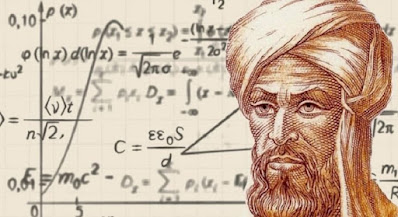Muhammad Ibnu Musa Al-Khawarizm

Al-Khwarizmi also used it to create a theory of algebra in the 9th century. Apart from that, he also developed a method for multiplying and dividing numbers called algorithms.

In the past, before Al-Khwarizmi introduced the number zero, scientists used a kind of list that distinguished units, tens, hundreds, thousands, and so on. The list known as the abacus serves to keep each number in the number from being confused with each other from their place or position in the count.

This system lasted until the 12th century AD, when Western scientists began to choose to use raqm al-binji (Arabic numerals) in their number system. Raqm albinji uses the number "zero" adopted from Indian numerals, providing a decimal numbering system that has never been used before.

At the beginning of extracting the concept of the number zero from books from India, Al Khwarizmi wrote down his efforts and findings in the book Al Khwarizmi on the Hindu Art of Reckoning. The book by Al Khwarizmi describes the Indian number placement system based on 1, 2, 3, 4, 5, 6, 7, 8, 9, and 0.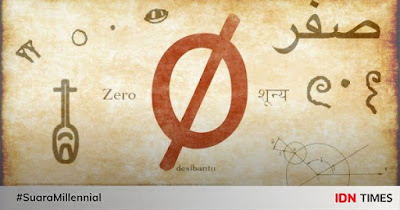Shifr is the number 0 ancient arabic nation

The number zero is described by Al Khwarizmi for its role in computing systems and number placement systems in Al-Khwarizmi's book, al-Jabr wa al-Muqabalah in 773 AD. , as quoted from the research of M Kharis Madjid, "Zero as a Muslim Contribution to Modern Mathematics" in the Journal of the Study of Religions and Islamic Thought.

The book was translated in London in 1831 by the English mathematician, Fredrick Rosen, and subsequently edited into Arabic in 1939 by two Egyptian mathematicians, Ali Mustafa Musyarrafa and Muhammad Mursi Ahmad. Previously, in the 12th century, this work was also translated by a mathematician from Chester, England, Robert (Latin: Robertus Castrensis), with the title Liber Algebras et Al-mucabola.

through his first book, Al-Mukhtasar fi Hisab al-Jabr wa al-Muqabalah (Summary of Algebraic and Comparative Calculations), Al-Kha warizmi introduced the number zero which in Arabic is called shifr. The monumental work also discusses systematic solutions of linear and quadratic notation.

5. Known in Europe in the 12th century

The number zero only arrived in Europe around the 12th century. Brought by the Muslim Moors from Morocco when they conquered Andalusia. However, there was a conflict among Europeans. Some think that zero or "sifr" is a devil's number or a code that endangers the country. In fact, the Italian government even banned numbers from India-Arabic from being used, lho.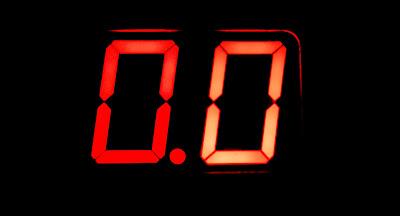Number Digital

Fortunately, an Italian mathematician, Leonardo Fibonacci created the Fibonacci Theory involving the number zero. This theory later became popular and was used to help prepare books for traders. Although it should be used secretly, this figure is still used in Europe. The name "sifr" also evolved into "cipher", which also means "password" or "code".

It wasn't until the 1600s that the free zero became widely used throughout Europe. European scientists such as Rene Descartes created a Cartesian coordinate system. As well as Isaac Newton, and Gottfried Wilhem Leibniz succeeded in developing calculus. In turn, calculus paved the way for the development of physics, engineering, computers, and financial and economic theory.

6. The Current Role of the Number 0 in the World

In the 17th century, the number zero reached its peak when it was used as the basis for the Cartesian coordinates (x and y graphs) discovered by the French philosopher Descartes. His system is still used today in any field, from engineering to computer graphics.

Some even say: "The Renaissance era was really made bright by Arabic numbers, including zero. As a result, the arithmetic world that used to seem only black and white, is now full of colour.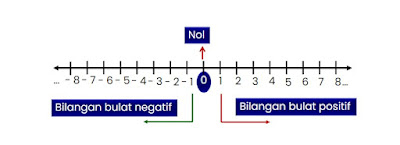Koordinat KartesiusWith the increasingly strong role of the number zero, another interesting thing emerges from zero, namely its division of other zeros which are often considered complicated. This became the basis of one of the favorite areas of mathematics, namely calculus. Calculus is full of probabilities that mathematicians can use to predict what will happen in the futureFrom then on, the number zero spread to all corners of the world and sticks with human calculations to this day."It turns out that the story behind the number 0 is full of twists and turns and a very long story, so it can be recognized and used as one of the current numbers."

### Blog authors